LearnFast.Ninja

# Insert math symbols in Word 2010

This tutorial show how to insert math symbol like: equation, fraction, script, radical, integral, function, bracket, limit and log,operator, matrix, linear, division sing, infinity, approximately, less then, not equal to, greater then etc..

Step 1: Click on Insert tab then click on Equation then click Insert New Equation .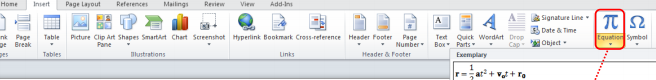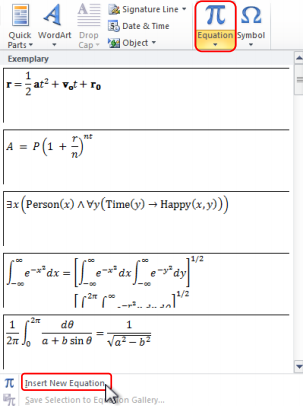Result: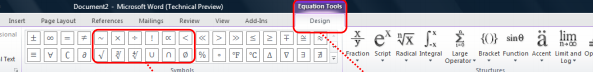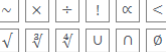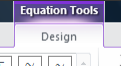Tags

word